# Q3. Fill in the following blanks with the correct answer (7 Marks) 1. Which of the...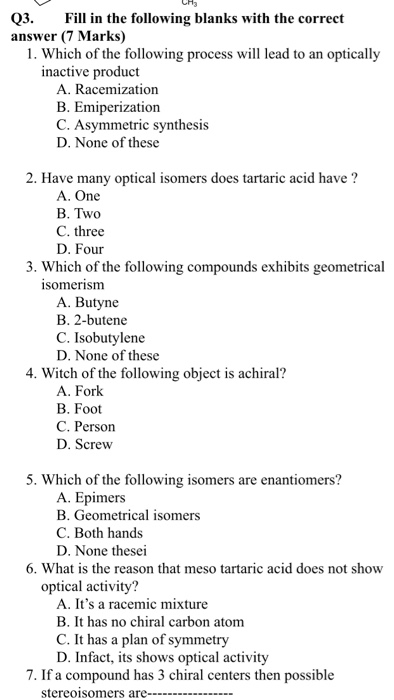Q3. Fill in the following blanks with the correct answer (7 Marks) 1. Which of the following process will lead to an optically inactive product A. Racemization B. Emiperization C. Asymmetric synthesis D. None of these 2. Have many optical isomers does tartaric acid have ? A. One B. Two C. three D. Four 3. Which of the following compounds exhibits geometrical isomerism A. Butyne B. 2-butene C. Isobutylene D. None of these 4. Witch of the following object is achiral? A. Fork B. Foot C. Person D. Screw 5. Which of the following isomers are enantiomers? A. Epimers B. Geometrical isomers C. Both hands D. None thesei 6. What is the reason that meso tartaric acid does not show optical activity? A. It's a racemic mixture B. It has no chiral carbon atom C. It has a plan of symmetry D. Infact, its shows optical activity 7. If a compound has 3 chiral centers then possible stereoisomers are-
Show transcribed image text

This Homework Help Question: "Q3. Fill in the following blanks with the correct answer (7 Marks) 1. Which of the..." No answers yet.

We need 10 more requests to produce the answer to this homework help question. Share with your friends to get the answer faster!

0 /10 have requested the answer to this homework help question.

Once 10 people have made a request, the answer to this question will be available in 1-2 days.
All students who have requested the answer will be notified once they are available.
##### Add Answer of: Q3. Fill in the following blanks with the correct answer (7 Marks) 1. Which of the...
Similar Homework Help Questions
• ### Which of the following process will lead to an optically inactive product A. Racemization B. Emiperization...

Which of the following process will lead to an optically inactive product A. Racemization B. Emiperization C. Asymmetric synthesis D. None of these

• ### Which of the following is a true statement? A. All achiral molecules are meso.

Which of the following is a true statement? A. All achiral molecules are meso. b. All chiral molecules possess a plane of symmetry. c. All molecules which posses a single asymmetric center of the S configuration are levorotatory . D. A mixture of achiral compounds will be optically inactive. E. All molecules which possess 2 or more chirality centers will be chiral.

• ### 10) Which of the following statements is (are) true for the compound (R)-2-butanol? A) This compound...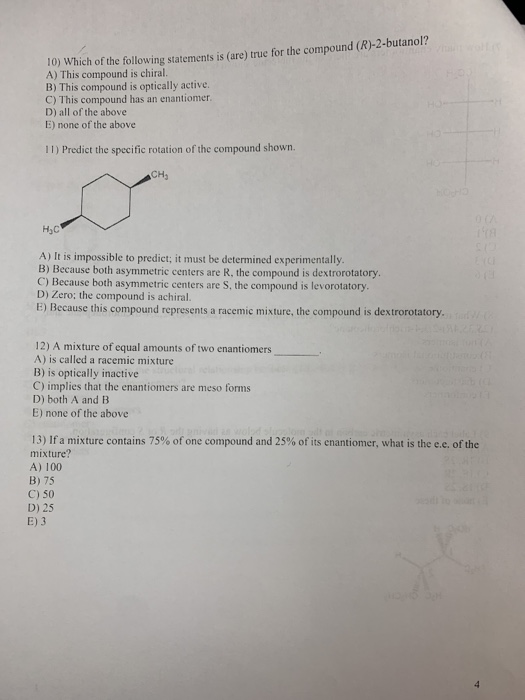10) Which of the following statements is (are) true for the compound (R)-2-butanol? A) This compound is chiral. B) This compound is optically active. C) This compound has an enantiomer D) all of the above E) none of the above 11) Predict the specific rotation of the compound shown. CH нс A) It is impossible to predict; it must be determined experimentally. B) Because both asymmetric centers are R, the compound is dextrorotatory. C) Because both asymmetric centers are S,...

• ### 8. Indicate whether each of the following statements is true or false. If a statement is...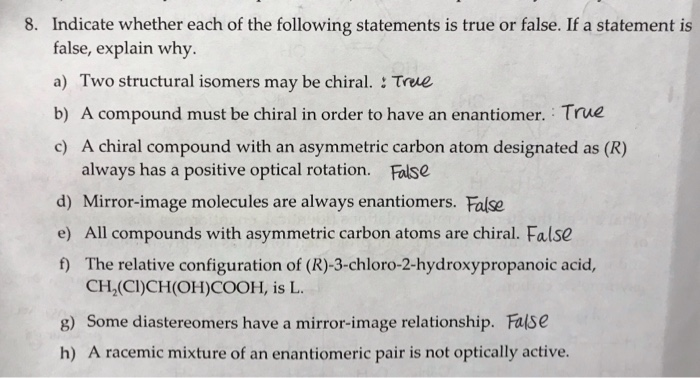8. Indicate whether each of the following statements is true or false. If a statement is false, explain why a) Two structural isomers may be chiral. : True b) A compound must be chiral in order to have an enantiomer. True c) A chiral compound with an asymmetric carbon atom designated always has a positive optical rotation. False (R) as d) Mirror-image molecules are always enantiomers. False chiral. False e) All compounds with asymmetric carbon atoms are f)The relative configuration...

• ### 9. C (Z-2-hexene d. They are all of equal stability. Choose the most stable alkene among...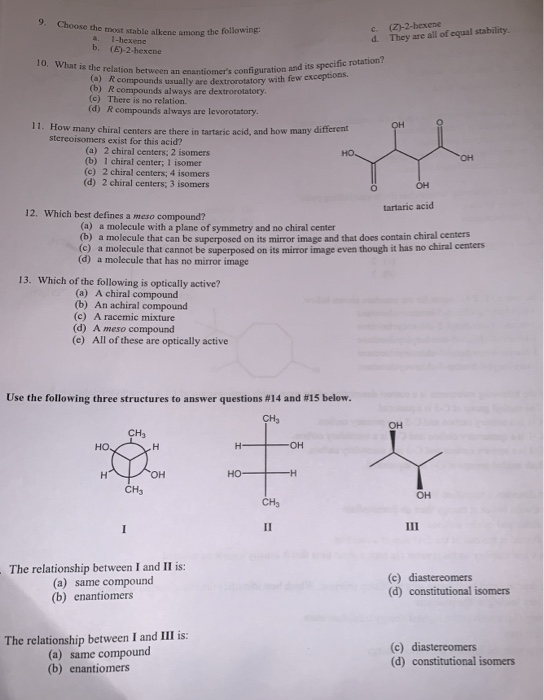9. C (Z-2-hexene d. They are all of equal stability. Choose the most stable alkene among the following a 1-hexene b. (E)-2-hexene 10. What is the relation between an enantiomer's configuration and its specific rotation? (a) Rcompounds usually are dextrorotatory with few exceptions (b) R compounds always are dextrorotatory. (c) There is no relation. (d) R compounds always are levorotatory 11. How many chiral centers are there in tartaric acid, and how many different stereoisomers exist for this acid? он...

• ### A. Consider the following pairs of structures. Designate each chirality center as (R) or (S) and...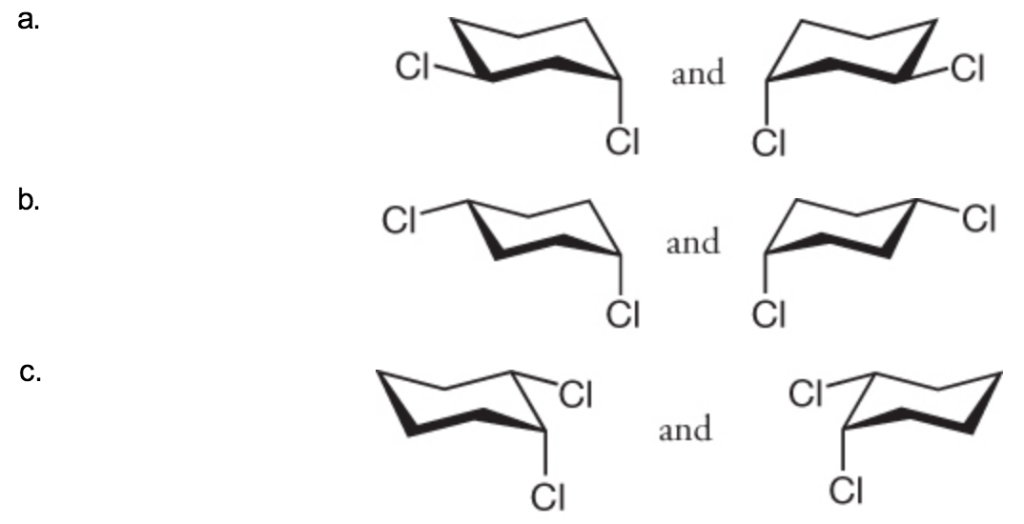A. Consider the following pairs of structures. Designate each chirality center as (R) or (S) and identify the relationship between them by describing them as representing enantiomers, diastereomers, constitutional isomers, or two molecules of the same compound. Use handheld molecular models to check your answers. B.  Tartaric acid [HO2CCH(OH)CH(OH)CO2H] was an important compound in the history of stereochemistry. Two naturally occurring forms of tartaric acid are optically inactive. One optically inactive form has a melting point of 210 − 212 °...

• ### the answers i circled are incorrect. can you give me the correct answers and explain why?...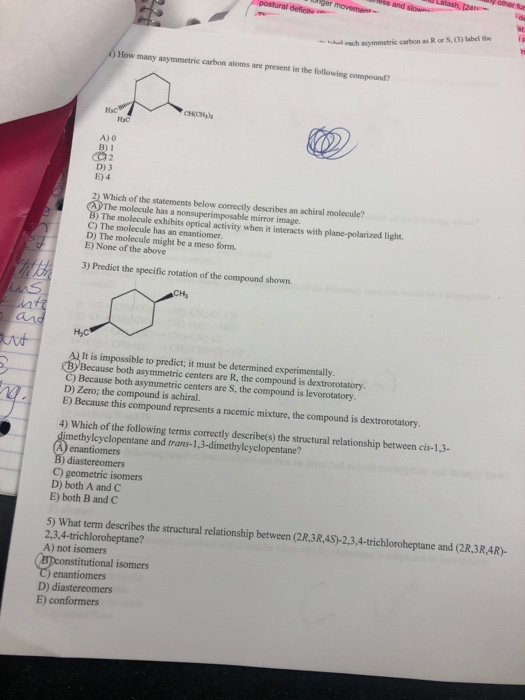the answers i circled are incorrect. can you give me the correct answers and explain why? postural de Pero ss and so Lalash, an walach asymmetric carbon as Ror S. (3) label the How many asymmetric carbon atoms are present in the following compound A) B) 1 D) 3 E) 4 2) Which of the statements below correctly describes an achiral molecule? The molecule has a nonsuperimposable mirror image. B) The molecule exhibits optical activity when it interacts with plane-polarized...

• ### Which of the following structures is most stable? 2) How many stereoisomers does the molecule below...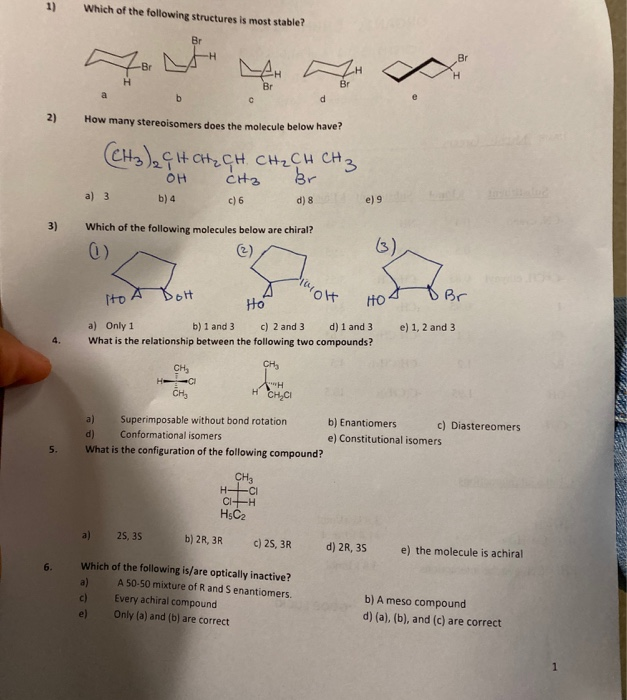Which of the following structures is most stable? 2) How many stereoisomers does the molecule below have? (СН), С+ снен снаси сна OH CH₃ br. a) 3 6) 4 c) 6 d) 8 e 9 3) Which of the following molecules below are chiral? 0) to A Boh A MOH HOS DBr e) 1, 2 and 3 a) Only 1 b) 1 and 3 c) 2 and 3 d) 1 and 3 What is the relationship between the following two...

• ### the answers i circled are incorrect. can you give me all the correct answers and explain...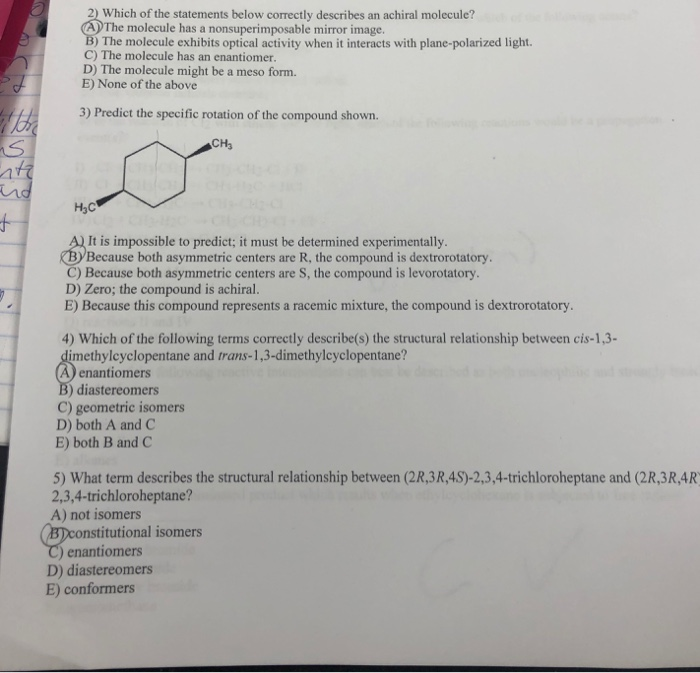the answers i circled are incorrect. can you give me all the correct answers and explain why? 2) Which of the statements below correctly describes an achiral molecule? A The molecule has a nonsuperimposable mirror image. cule exhibits optical activity when it interacts with plane-polarized light. C) The molecule has an enantiomer. D) The molecule might be a meso form. E) None of the above 3) Predict the specific rotation of the compound shown. CH нс 4 A) It is...

• ### HELP tatMtiele Cheice 2.0peints.each Which of the fellowing structures is mest stable? How many stereaisomers does...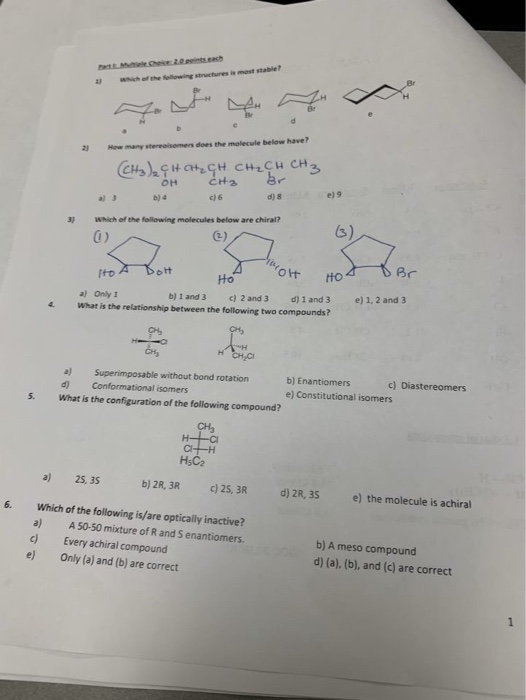HELP tatMtiele Cheice 2.0peints.each Which of the fellowing structures is mest stable? How many stereaisomers does the molecule below have? 2) CH)HCtCH CHCH CH3 CH2 br OH e) 9 d) 8 c) 6 b)4 al 3 Which of the follewing molecules below are chiral? 3) HO Ho e) 1, 2 and 3 a) Only 1 d) 1 and 3 b) 1 and 3 c) 2 and 3 What is the relationship between the following two compounds? 4 сн, H- H...

Free Homework App